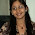### Geometry Problem : Line of Sight

Source: IBM Ponder This November 2012

Problem:A gardener plants a tree on every integer lattice point, except the origin, inside a circle with a radius of 9801. The trees are cylindrical in shape and all grow together at the same rate.

As the trees grow, more and more points outside the circle of trees stop having a direct line of sight with the origin. What will be the trees' radius when the origin first loses its line of sight with all the points outside the circle? Please give your answer as a decimal number with an accuracy of 13 digits (13 significant digits).

The image is a sketch of a forest of radius 5 and a light beam entering the origin (center of the forest).

1.Line of sight between the point A = (70,69) and B = (70,70) passing through (0,0)and equidistant from both A and B. Will be the last standing line of sight.

2.No the above solution is wrong

Line of sight between the point A = (1,0) and B = (98,1) passing through (0,0)and equidistant from both A and B. Will be the last standing line of sight.

I did a miscalculation

3.can you please explain how you arrived at those two points

4.Inteersting post but i am not getting the answer for this puzzle.. Abacus may solve it but how it may implement..Please help me to know.

5.Answer as per IBM Ponder This:

The answer is 1/sqrt(d), where d is the smallest integer satisfying d=a^2+b^2, where a is co-prime to b, and d>=R^2.
Clearly, d<=R^2+1 by choosing a=R and b=1; and d can be R if and only if all the prime factors of R are 1 modulo 4.
In our case, R=9801=3^4*11^2, so the critical radius is 1/sqrt(R^2+1) which is 0.000102030404529629...

Can anyone decipher the solution?